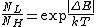# absolute zero

(redirected from 0 Kelvin)
Also found in: Dictionary, Thesaurus, Medical.

## absolute zero

absolute zero, the zero point of the ideal gas temperature scale, denoted by 0 degrees on the Kelvin and Rankine temperature scales, which is equivalent to −273.15℃ and −459.67℉. For most gases there is a linear relationship between temperature and pressure (see gas laws), i.e., gases contract indefinitely as the temperature is decreased. Theoretically, at absolute zero the volume of an ideal gas would be zero and all molecular motion would cease. In actuality, all gases condense to solids or liquids well above this point. Although absolute zero cannot be reached, temperatures within a few billionths of a degree above absolute zero have been achieved in the laboratory. At such low temperatures, gases assume nontraditional states, the Bose-Einstein and fermionic condensates. See also low-temperature physics; temperature.

## Absolute zero

The temperature at which an ideal gas would exert no pressure. The Kelvin scale of temperatures is defined in terms of the triple point of water, T3 = 273.16° (where the solid, liquid, and vapor phases coexist), and absolute zero. Temperature is measured most simply via the constant-volume ideal-gas thermometer, in which a small amount of gas is introduced (in order to limit the effect of interactions between molecules) and then sealed off, and the gas pressure P referenced to its value at the triple point P(T3) is measured. The ideal-gas law applies if the molecules in a gas exert no forces on one another and if they are not attracted to the walls. Absolute zero is the temperature at which the pressure of a truly ideal gas would vanish. See Temperature measurement

According to classical physics, all motion would cease at absolute zero; however, the quantum-mechanical uncertainty principle requires that there be a small amount of residual motion (zero-point motion) even at absolute zero. See Kinetic theory of matter, Uncertainty principle

Temperature can also be defined from the Boltzmann distribution. If a collection of spin-1/2 magnetic ions is placed in a magnetic field, the ratio of the occupancy of the lower to the higher energy state is given by the equation below.Here k is Boltzmann's constant, ΔE is the magnitude of the difference in energy between the states, and T is the Kelvin temperature. Thus, at high temperatures the two states have nearly equal occupation probability, while the lower energy state is progressively favored at lower temperatures. At absolute zero, only the lower energy level is occupied. This relation allows for the possibility of negative temperatures when the population of the higher energy state exceeds that of the lower state. See Boltzmann constant, Boltzmann statistics

Negative temperatures notwithstanding, the third law of thermodynamics states that the absolute zero of temperature cannot be attained by any finite number of steps. The lowest (and hottest) temperatures that have been achieved are on the order of a picokelvin (10-12K). These are spin temperatures of nuclei which are out of equilibrium with the lattice vibrations and electrons of a solid. The lowest temperatures to which the electrons have been cooled are on the order of 10 microkelvins in metallic systems. See Low-temperature physics, Temperature

McGraw-Hill Concise Encyclopedia of Physics. © 2002 by The McGraw-Hill Companies, Inc.

## absolute zero

The zero value of the thermodynamic temperature scale, i.e. 0 K (–273.15 °C). Absolute zero is the lowest temperature theoretically possible. At absolute zero molecular motion almost ceases.
Collins Dictionary of Astronomy © Market House Books Ltd, 2006
The following article is from The Great Soviet Encyclopedia (1979). It might be outdated or ideologically biased.

## Absolute Zero

the point from which absolute temperatures are measured; it lies at 273.16°K below the temperature of the triple point of water. The existence of absolute temperature and of absolute zero follows from the second law of thermodynamics; the third law of thermodynamics indicates that absolute zero cannot be attained. As the temperature approaches absolute zero, the thermal characteristics of matter such as the entropy, heat capacity, and coefficient of thermal expansion tend to zero. The sharp drop in the intensity of the thermal motion of atoms and molecules near absolute zero results in the ordered crystalline structure that all substances, with the exception of liquid helium, have under these conditions.

According to classical physics, at absolute zero the energy of thermal (random) motion of molecules and atoms in a substance is equal to zero. According to quantum mechanics, however, at absolute zero the atoms or molecules at sites in a crystalline lattice are not completely at rest but undergo “zero” vibrations and have so-called zero energy. If the atomic mass and the interaction energy between atoms are very small, the zero vibrations may hinder the formation of a crystalline lattice. This is the case in the helium isotopes 3He and 4He, which remain liquid right down to the lowest attainable temperatures.

Obtaining temperatures that are as close as possible to absolute zero is a complicated experimental problem, but temperatures have been reached which are within millionths of a degree of absolute zero.

## absolute zero

[′ab·sə‚lüt ′zir·ō]
(thermodynamics)
The temperature of -273.16°C, or -459.69°F, or 0 K, thought to be the temperature at which molecular motion vanishes and a body would have no heat energy.
McGraw-Hill Dictionary of Scientific & Technical Terms, 6E, Copyright © 2003 by The McGraw-Hill Companies, Inc.

## absolute zero

the lowest temperature theoretically attainable, at which the particles constituting matter would be in the lowest energy states available; the zero of thermodynamic temperature; zero on the International Practical Scale of Temperature: equivalent to --273.15°C or --459.67°F
Collins Discovery Encyclopedia, 1st edition © HarperCollins Publishers 2005

## absolute zero

The temperature at which molecular activity is at a minimum. Absolute zero is -273.15 degrees Celsius and -459.67 degrees Fahrenheit. See Kelvin.
Copyright © 1981-2019 by The Computer Language Company Inc. All Rights reserved. THIS DEFINITION IS FOR PERSONAL USE ONLY. All other reproduction is strictly prohibited without permission from the publisher.
Site: Follow: Share:
Open / Close